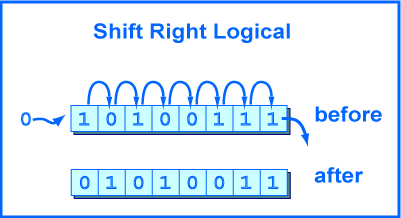shiftless

# Shift Right LogicalMIPS also has a shift right logical instruction. It moves bits to the right by a number of positions less than 32. The high-order bit gets zeros and the low-order bits are discarded.

If the bit pattern is regarded as an unsigned integer, or a positive two's comp. integer, then a right shift of one bit position performs an integer divide by two. A right shift by N positions performs an integer divide by 2N.

The "trick" of dividing an integer by shifting should not be used in place of the MIPS arithmetic divide instruction (which will be covered in a few chapters). If you mean "divide" that is what you should write. But the trick is often used in hardware, and sometimes pops up in odd software uses, so you should know about it.

```srl  d,s,shft      # \$d gets the logical
# right shift of \$s
# by shft positions.
# shft is a 5-bit
# integer, 0 ≤ shft < 32
```

### QUESTION 6:

(Trick Question:) What is the net result if a bit pattern is logical left shifted 2 positions and then logical right shifted 2 positions?